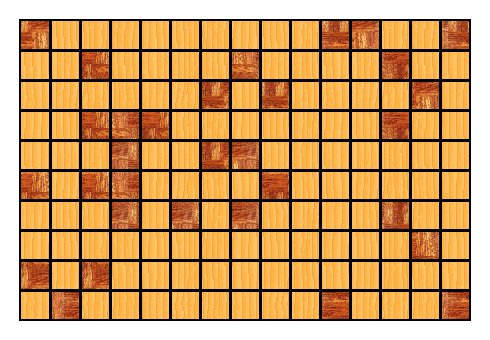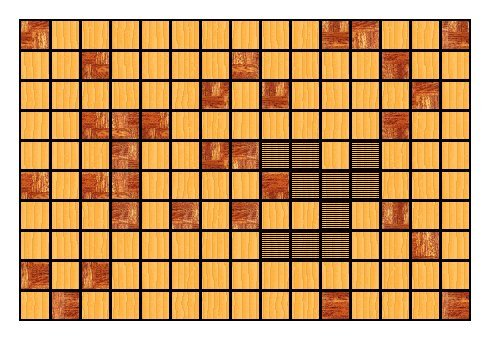### Problem Statement

You own a small wood-cutting business and would like to organize the operations to get the maximum profit.

You have got a large rectangular plate of wood divided into 1x1 squares. The wood in the most of squares is fine, however in some squares it is of bad quality and therefore they are unusable. The state of material in your plate is described by a String[] plate, where each '.' character represents a square with fine wood and each 'X' character is an unusable square.

You are going to receive several orders to cut different figures from the wood. The amount of orders orderCnt is known in advance, however the details of each order are unknown until you receive it. These details are:
• The figure to be cut. It will be a set of K 4-connected squares (see notes). In order to describe the figure, a String[] figure will be used, where each 'X' character corresponds to a figure's square, while all other characters are '.' and can be ignored.
• An income that you will get for satisfying the order.
You will receive the orders one at a time and for each order you need to decide whether you accept or reject it before receiving the next order. If you accept the order, then you need to cut the required figure. In other words, you should choose K squares on the plate such that: they are not cut yet, the wood is in a good state there and they form exactly the same figure as required. You are allowed to rotate the requested figure, and also you're allowed to reflect it horizontally and/or vertically. After the appropriate squares are chosen, the figure is cut of them and they can't be used for producing other figures anymore.

Your code should implement two methods:
• int init(String[] plate, int K, int orderCnt). This method is called once per test case to give you the initial state of the plate, the number of squares in each requested figure and the number of orders. The return from this method is ignored.
• int[] processOrder(String[] figure, int income). This method will be called once for each received order to give you the figure that is requested and the income you can get for accepting the order. If you would like to reject this order, just return an empty int[]. Otherwise, you must return the int[] consisting of 5 elements (in this exact order):
1. The number of times the figure is to be rotated clockwise before being cut (0, 1, 2 or 3). It is assumed that all rotations are done before reflections.
2. The number of times the figure is to be reflected vertically (0 or 1).
3. The number of times the figure is to be reflected horizontally (0 or 1).
4. The number of the topmost row (0-based, starting from the top row) among the squares chosen from plate for cutting the figure.
5. The number of the leftmost column (0-based, starting from the left column) among the squares chosen for cutting the figure.
For example, you have the following plate where dark squares are of bad quality and light squares are fine:If you received an order to cut a figure as on picture below, then you can, for example, choose to rotate it once clockwise and then to reflect it horizontally. The result will be the following:You can then choose to cut the figure from the following squares:In this case, your return from processOrder will be {1, 0, 1, 4, 8}.

Each test case will be generated as follows:
• Each of the number of rows N and the number of columns M in plate will be chosen as a random integer between 10 and 100, inclusive (uniform distribution is used here and later unless other distribution is specified).
• A random integer P between 0 and 30, inclusive, will be generated. Each square will be initially of bad quality with probability P%.
• K will be between 3 and 10, inclusive.
• orderCnt will be between (N*M)/2 and N*M, inclusive.
• figure in each order will be generated as follows. All possible 4-connected figures of K squares will be generated (equal figures with respect to rotations/reflections will be considered the same). For each of the figures, it's weight will be generated as a random integer between 1 and 100, inclusive. Each figure can be chosen for an order with the probability proportional to its weight. The choice for each order is done independently.
• income in each order will be between 1 and 100, inclusive.
Your income is the total income you get from all accepted orders. The score for a test case will be defined as YOUR_INCOME / MAXIMUM_INCOME, where MAXIMUM_INCOME is the maximum income that have been obtained for this test case by any of the competitors.

A visualization tool is provided for offline testing.

### Definition

 Class: CuttingFigures Method: init Parameters: String[], int, int Returns: int Method signature: int init(String[] plate, int K, int orderCnt) Method: processOrder Parameters: String[], int Returns: int[] Method signature: int[] processOrder(String[] figure, int income) (be sure your methods are public)

### Notes

-If your return from any call of processOrder is invalid, the execution of your solution will be terminated immediately. It can be invalid if wrong number of elements is returned (not 0 or 5), wrong number of rotations or reflections is specified, or the figure can't be cut at the specified place of the plate (some square is outside the plate, is already cut or contains wood of bad quality).
-Two squares are 4-neighbours if they share a side. Two squares are 4-connected if there exists a path starting at one of them, ending at the other and such that consecutive squares in the path are 4-neighbours. A set of squares is 4-connected if every two squares from the set are 4-connected.
-The memory limit is 1024 MB and the time limit is 20 seconds (which includes only time spent in your code). There is no explicit code size limit.
-If your solution is terminated before all orders are processed (due to invalid return, time limit, memory limit, etc.), your score will be based on the income collected before termination.
-There are 10 example test cases and 100 full submission test cases.

### Constraints

-Each test case will be generated as explained in the statement.
-For each order, there will be no rows or columns in figure entirely consisting of '.' characters.

### Examples

0)

 `"1"`
```Returns:
"seed = 1<br/><br/>89x54 plate, 17% for a square to be of bad quality.<br></br><br></br>4717 orders, K = 7."```
1)

 `"2"`
```Returns:
"seed = 2<br/><br/>23x50 plate, 27% for a square to be of bad quality.<br></br><br></br>1015 orders, K = 5."```
2)

 `"3"`
```Returns:
"seed = 3<br/><br/>99x29 plate, 0% for a square to be of bad quality.<br></br><br></br>2268 orders, K = 10."```
3)

 `"4"`
```Returns:
"seed = 4<br/><br/>52x83 plate, 23% for a square to be of bad quality.<br></br><br></br>3093 orders, K = 6."```
4)

 `"5"`
```Returns:
"seed = 5<br/><br/>83x59 plate, 17% for a square to be of bad quality.<br></br><br></br>3406 orders, K = 10."```
5)

 `"6"`
```Returns:
"seed = 6<br/><br/>34x36 plate, 23% for a square to be of bad quality.<br></br><br></br>978 orders, K = 9."```
6)

 `"7"`
```Returns:
"seed = 7<br/><br/>98x54 plate, 7% for a square to be of bad quality.<br></br><br></br>3986 orders, K = 4."```
7)

 `"8"`
```Returns:
"seed = 8<br/><br/>49x30 plate, 27% for a square to be of bad quality.<br></br><br></br>860 orders, K = 10."```
8)

 `"9"`
```Returns:
"seed = 9<br/><br/>55x74 plate, 22% for a square to be of bad quality.<br></br><br></br>3277 orders, K = 8."```
9)

 `"10"`
```Returns:
"seed = 10<br/><br/>84x41 plate, 19% for a square to be of bad quality.<br></br><br></br>3386 orders, K = 8."```

#### Problem url:

http://www.topcoder.com/stat?c=problem_statement&pm=11122

#### Problem stats url:

http://www.topcoder.com/tc?module=ProblemDetail&rd=14355&pm=11122

Unknown

Simulation